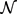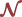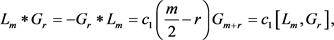﻿ 超Virasoro代数上的Poisson超结构

超Virasoro代数上的Poisson超结构Poisson Algebra Structures on the Super-Virasoro Algebra

Abstract: Poisson algebras are algebras with an algebra structure and a Lie algebra structure, both of which satisfy the Leibniz law. Super Virasoro algebra is a kind of infinite dimensional Lie superalgebra, which plays an important role in the quantum field theory. This paper mainly studies the Poisson structure on the super Virasoro algebra.

1. 引言

Virasoro代数是一个特征零域上的无限维李代数，在二维共形量子场理论中具有重要作用，受到数学家和物理学家的关注。超Virasoro代数，也称作N = 1超共形代数(见  )，可以看成是Virasoro代数的非平凡Z2扩张。这样的扩张主要有两种，它们分别是Ramond代数和Neveu-Schwarz代数。这两类代数在超弦理论和超共形场理论中有着密切的联系和重要的应用(见   )。确定超Virasoro代数上的其它代数结构问题是李代数研究中比较有意义的一项工作，论文  和  分别研究了超Virasoro代数上的左超对称代数结构和李超双代数结构。

Poisson代数源于Poisson几何的研究，具有代数结构和李代数结构，乘法与李代数乘法之间满足Leibniz法则。近来许多人研究了结合的Poisson代数结构问题，如姚裕丰等研究了Witt代数和Virasoro代数上的Poisson代数结构  ，靳全勤和佟洁在文   中研究了Toroidal李代数等代数上的结合Poisson代数结构。

2. 预备知识

$\begin{array}{l}\left[{L}_{m},{L}_{n}\right]=\left(m-n\right){L}_{n+m}+\frac{1\text{}}{12}\left({m}^{3}-m\right){\delta }_{m+n,0}C,\\ \left[{L}_{m},{G}_{r}\right]=\left(\frac{m}{n}-r\right){G}_{m+r},\\ \left[{G}_{r},{G}_{s}\right]=2{L}_{r+s}+\frac{1}{12}\left(4{r}^{2}\text{}-\text{}1\right){\delta }_{r+s,0}C,\\ \left[{N}_{\stackrel{¯}{0}},C\right]=\left[{N}_{\stackrel{¯}{1}},\text{}C\right]=0,\text{}\forall n,m,r,s\in ℤ\end{array}$$\left(1-\epsilon \right)ℤ$ -阶化的： $\mathcal{N}={\otimes }_{i\in \left(1-\epsilon \right)ℤ}{\mathcal{N}}_{i}$ 。当 $\epsilon =0$ 时， ${\mathcal{N}}_{i}=\text{span}\left\{{L}_{i},{G}_{i},{\delta }_{i,0}C\right\}$ ；当 $\epsilon =\frac{1}{2}$ 时，对任意 $i\in ℤ$${\mathcal{N}}_{i}=spa{n}_{ℂ}\left\{{L}_{i}\text{,}{\delta }_{i,0}C\right\}$${\mathcal{N}}_{i+\frac{1}{2}}=spa{n}_{ℂ}\left\{{G}_{i+\frac{1}{2}}\right\}$ 。其Cantar子代数为 $\mathcal{H}=ℂ{L}_{0}+ℂC$

$\mathcal{S}$$\mathcal{N}$ 关于理想 $ℂ\left\{C\right\}$ 的商代数，则 $\mathcal{N}$ 为李超代数 $\mathcal{S}$ 的普遍中心扩张  。

$\left[a,b\ast c\right]=\left[a,b\right]\ast c+{\left(-1\right)}^{|a||b|}b\ast \left[a,c\right],\text{\hspace{0.17em}}\forall a,b,c\in A.$ (2.1)

${L}_{m}\ast {L}_{n}=-{c}_{1}\left(n-m\right){L}_{m+n}-\frac{{m}^{3}-m\text{}}{12}{\delta }_{m+n,0}{c}_{1}C=-{c}_{1}\left[{L}_{m},{L}_{n}\right],$ (2.2)

3. 李超代数 $\mathcal{S}$ 上的Poisson超代数结构

${\mathcal{S}}_{i}\ast {\mathcal{S}}_{j}\subseteq {\mathcal{S}}_{i+j},\forall i,j\in ℤ$ .

$\left[{L}_{0},x\ast y\right]=\left[{L}_{0},x\right]\ast y+x\ast \left[{L}_{0},y\right]=-ix\ast y-jx\ast y=-\left(i+j\right)x\ast y.$

$x\ast y\in {\mathcal{S}}_{i+j}$ 。因此对任意的 $i,j\in ℤ$ ，都有 ${\mathcal{S}}_{i}\ast {\mathcal{S}}_{j}\subseteq {\mathcal{S}}_{i+j}$

$\begin{array}{l}{L}_{m}\ast {G}_{r}=-{G}_{r}\ast {L}_{m}={c}_{1}\left(m-2r\right){G}_{m+r},\\ {G}_{r}\ast {G}_{s}=2{c}_{1}{L}_{r+s}\end{array}$

$\begin{array}{l}{L}_{m}\ast {L}_{n}={c}_{1}\left(m-n\right){L}_{m+n},\\ {L}_{m}\ast {G}_{r}={a}_{m,r}{G}_{m+r},\\ {G}_{r}\ast {L}_{m}={b}_{r,m}{G}_{m+r},\\ {G}_{r}\ast {G}_{s}={c}_{r,s}{L}_{r+s}\end{array}$

$\left(\frac{k}{2}-r-m\right){a}_{m,r}=\left(k-m\right){a}_{m+k,r}+\left(\frac{k}{2}-r\right){a}_{m,r+k}.$ (3.1)

$\frac{{a}_{m,r}}{r-\frac{m}{2}}=\frac{{a}_{m,r}{}_{+m}}{r+m-\frac{\text{}m}{2}}={c}_{2},$

${a}_{m,r}={c}_{2}\left(r-\frac{m}{2}\right),$ (3.2)

$\left(\frac{k}{2}-r-m\right){b}_{r,m}=\left(\frac{k}{2}-r\right){b}_{r+k,m}+\left(k-m\right){b}_{r,m+k},$ (3.3)

$\frac{{b}_{r,m}}{r-\frac{m}{2}}=\frac{{b}_{r+m,m}}{r+m-\frac{m}{2}}={c}_{3},$

${b}_{r,m}={c}_{3}\left(r-\frac{m}{2}\right),$ (3.4)

$\left(\frac{r+s}{2}-k\right){c}_{r,s}=2{b}_{r,k+s}-2{a}_{k+r,s},$ (3.5)

${c}_{r,s}=2{c}_{3}=-2{c}_{2}.$ (3.6)

$\left(\frac{m}{2}-k\right){c}_{m+k,r}=-2{a}_{m,r}+2{c}_{1}\left(m-k-r\right).$ (3.7)

${c}_{1}=-{c}_{2}={c}_{3}.$

$\begin{array}{l}{L}_{m}\ast {G}_{r}=-{G}_{r}\ast {L}_{m}={c}_{1}\left(\frac{m}{2}-r\right){G}_{m+r},\\ {G}_{r}\ast {G}_{s}=2{c}_{1}{L}_{r+s}\end{array}$

4. 超Virasoro代数上的Poisson超结构

${L}_{m}\ast {L}_{n}={c}_{1}\left(m-n\right){L}_{m+n}+\frac{{m}^{3}-m}{12}{\delta }_{m+n,0}{c}_{1}C={c}_{1}\left[{L}_{m},{L}_{n}\right],$ (4.1)(4.2)

${G}_{r}\ast {G}_{s}=2{c}_{1}{L}_{r+s}-\frac{4{r}^{2}-1}{12}{\delta }_{r+s,0}{c}_{1}C={c}_{1}\left[{G}_{r},{G}_{s}\right],$ (4.3)

${G}_{r}\ast {G}_{-r}={a}_{r}{L}_{0}+{c}_{r}C,$

$\left[{L}_{k},{G}_{r}{}_{-k}\ast {G}_{-r}\right]=\left[{L}_{k},{G}_{r-k}\right]\ast {G}_{-m}+{G}_{r-k}\ast \left[{L}_{k},{G}_{-r}\right],$

$\left\{\begin{array}{l}\left(\frac{3k}{2}-r\right){a}_{r}+\left(r+\frac{k}{2}\right){a}_{r-k}=4k{c}_{1}\\ \left(\frac{3k}{2}-r\right){c}_{r}+\left(r+\frac{\text{}k}{2}\right){c}_{r+\frac{k}{2}}=2\frac{{k}^{3}-k}{12}\end{array}$ (4.4)

${a}_{r}=2{c}_{1}\text{,}{c}_{r}=\frac{4{r}^{2}-1}{12}{c}_{1}.$

${G}_{r}\ast {G}_{-r}=2{c}_{1}{L}_{0}+\frac{4{r}^{2}-1}{12}{c}_{1}C.$

$C=2\left[{L}_{2},{L}_{-2}\right]-8{L}_{0}$

$\begin{array}{c}{G}_{r}\ast C=2{G}_{r}\ast \left[{L}_{2},{L}_{-2}\right]-8{G}_{r}\ast {L}_{0}\\ =2\left(\left[{L}_{2},{G}_{r}\ast {L}_{-2}\right]-\left[{L}_{2},{G}_{r}\right]\ast {L}_{-2}\right)-8{G}_{r}\ast {L}_{0}\\ =2{c}_{1}\left(r-3\right)\left(r+1\right){G}_{r}-2{c}_{1}\left(r+3\right)\left(r-1\right){G}_{r}+8{c}_{1}r{G}_{r}\\ =0\end{array}$

$C\ast {G}_{r}=2\left[{L}_{2},{L}_{-2}\right]\ast {G}_{r}-8{L}_{0}\ast {G}_{r}.$

$C\ast {G}_{r}=0.$

$C\ast C=0.$

 Yamanaka, I. and Sasaki, R. (1988) Super Virasoro Algebra and Solvable Supersymmetric Quantum Field Theories. Progress of Theoretical Physics, 79, 1167-1184.
https://doi.org/10.1143/PTP.79.1167

 Kac, V.G. (1997) Su-perconformal Algebra and Transitive Groups Actions on Quadrics. Communications in Mathematical Physics, 186, 233-252.
https://doi.org/10.1007/BF02885680

 Ademollo, M., et al. (1976) Supersymmetric Strings and Colour Confinement. Physics Letters B, 62, 105-110.
https://doi.org/10.1016/0370-2693(76)90061-7

 Kong, Xi. and Bai, C. (2008) Left-Symmetric Superalgebra Structures on the Super Virasoro Algebras. Pacific Journal of Mathematics, 235, 43-55.
https://doi.org/10.2140/pjm.2008.235.43

 Yang, H. (2009) Lie Super-Bialgebra Structures on Super-Virasoro Algebra. Frontiers of Mathematics in China, 4, 365-379.
https://doi.org/10.1007/s11464-009-0012-x

 姚裕丰. Witt代数和Virasoro代数上的Poisson代数结构[J]. 数学年刊, 2013, 34A(1): 111-128.

 靳全勤, 佟洁. To-roidal李代数上的Poisson代数结构[J]. 数学年刊, 2007, 28A(1): 57-70.

 佟洁, 靳全勤. 李代数的Poisson代数结构Ⅱ[J]. 数学杂志, 2010, 30(1): 145-151.

 Zusmanovich, P. (2013) A Compendium of Lie Structures on Tensor Products. Journal of Mathematical Science, 199, 266-288.
https://doi.org/10.1007/s10958-014-1855-6

 李雅南, 高寿兰, 刘东. 李代数W(2,2)上的Poisson结构[J]. 数学年刊A辑(中文版), 2016, 37(3): 267-272.

 赵晓晓, 高寿兰, 刘东. 扭Heisenberg-Virasoro代数上的Poisson结构[J]. 数学学报, 2016, 59(6): 775-782.

 Liu, D., Pei, Y. and Zhu, L. (2012) Lie Bialgebra Structures on the Twisted Heisenberg-Virasoro Algebra. Journal of Algebra, 359, 35-48.
https://doi.org/10.1016/j.jalgebra.2012.03.009

 Liu, D. and Pei, Y. (2019) Deformations on the Twisted Heisenberg-Virasoro Algebra. Chinese Annals of Mathematics, Series B, 40, 111-116.
https://doi.org/10.1007/s11401-018-0121-5

 Liu, D. and Jiang, C. (2018) Harish-Chandra Modules over the Twisted Heisenberg-Virasoro Algebra. Journal of Mathematical Physics, 49, Article ID: 012901.
https://doi.org/10.1063/1.2834916

 Liu, D. and Zhu, L. (2009) The Generalized Heisenberg-Virasoro Algebra. Frontiers of Mathematics in China, 4, 297-310.
https://doi.org/10.1007/s11464-009-0019-3

 Liu, D. (2016) Classification of Harish-Chandra Modules over Some Lie Algebras Related to the Virasoro Algebra. Journal of Algebra, 447, 548-559.
https://doi.org/10.1016/j.jalgebra.2015.09.035

Top